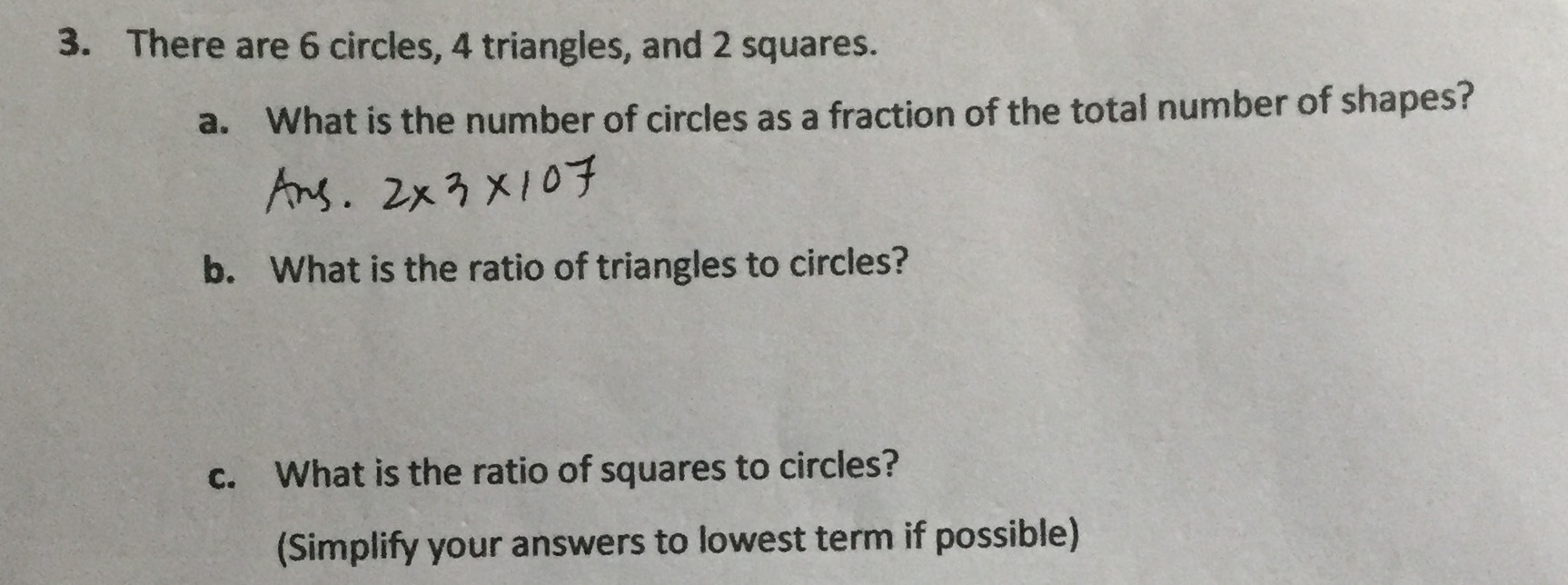### ¿Todavía tienes preguntas de matemáticas?

Pregunte a nuestros tutores expertos
Algebra
Pregunta3. There are $$6$$ circles, $$4$$ triangles, and $$2$$ squares. a. What is the number of circles as a fraction of the total number of shapes? b. What is the ratio of triangles to circles? c. What is the ratio of squares to circles? (Simplify your answers to lowest term if possible)

a. $$\frac{1}{2}$$